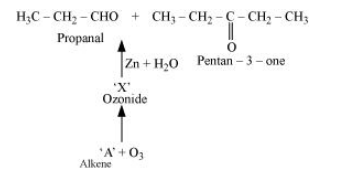# Obtain an expression for the mutual inductance between a long straight wire and a square loop of side a as shown in Fig. 6.21.

Question:

(a) Obtain an expression for the mutual inductance between a long straight wire and a square loop of side as shown in Fig. 6.21.

(b) Now assume that the straight wire carries a current of 50 A and the loop is moved to the right with a constant velocity, = 10 m/s.

Calculate the induced emf in the loop at the instant when = 0.2 m.

Take = 0.1 m and assume that the loop has a large resistance.Solution:

(a) Take a small element dy in the loop at a distance y from the long straight wire (as shown in the given figure).Magnetic flux associated with element $d y, d \phi=B d A$

Where,

dA = Area of element dy a dy

B = Magnetic field at distance y

$=\frac{\mu_{0} I}{2 \pi \mathrm{y}}$

I = Current in the wire

$\mu_{0}=$ Permeability of free space $=4 \pi \times 10^{-7} \mathrm{Tm} \mathrm{A}^{-1}$

$\therefore d \phi=\frac{\mu_{0} I a}{2 \pi} \frac{d y}{y}$

$\phi=\frac{\mu_{0} I a}{2 \pi} \int \frac{d y}{y}$

$y$ tends from $x$ to $a+x$.

$\therefore \phi=\frac{\mu_{0} I a}{2 \pi} \int_{x}^{a+x} \frac{d y}{y}$

$=\frac{\mu_{0} I a}{2 \pi}\left[\log _{e} y\right]_{x}^{a+x}$

$=\frac{\mu_{0} I a}{2 \pi} \log _{e}\left(\frac{a+x}{x}\right)$

For mutual inductance $M$, the flux is given as:

$\phi=M I$

$\therefore M I=\frac{\mu_{0} I a}{2 \pi} \log _{e}\left(\frac{a}{x}+1\right)$

$M=\frac{\mu_{0} a}{2 \pi} \log _{e}\left(\frac{a}{x}+1\right)$

(b) Emf induced in the loop, e $=B^{\prime} a v=\left(\frac{\mu_{0} I}{2 \pi x}\right) a v$

Given,

= 50 A

x = 0.2 m

a = 0.1 m

v = 10 m/s

$e=\frac{4 \pi \times 10^{-7} \times 50 \times 0.1 \times 10}{2 \pi \times 0.2}$

$e=5 \times 10^{-5} \mathrm{~V}$